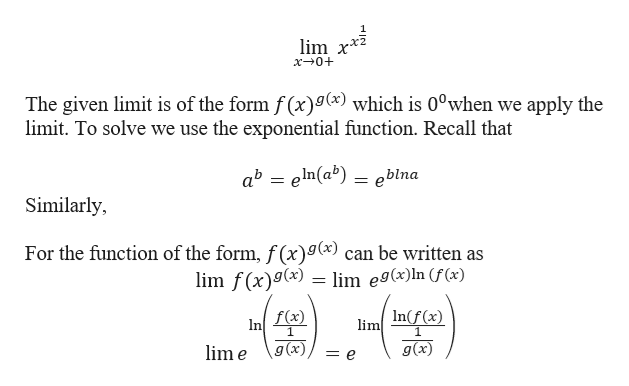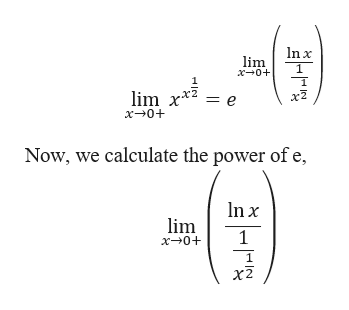Question
26 views

lim of xx^(1/2) as x approaches 0+

check_circle

Step 1

Given a function:

Step 2

We begin with applying the limit.help_outlineImage Transcriptioncloselim x* x 0+ The given limit is of the form f (x)9() which is 0°when we apply the limit. To solve we use the exponential function. Recall that ab eln(ab) eblna Similarly For the function of the form, f (x)g(x) can be written as lim f(x)g(x) lim eg(x)In (f(x) In(f (x) f(x) 1 lim In 1 g (x) g(x) lim e fullscreen
Step 3

Thus,

...help_outlineImage Transcriptionclosen x lim x 0+ = e lim x* x0+ Now, we calculate the power of e, lim x 0+ 1 x2 fullscreen

### Want to see the full answer?

See Solution

#### Want to see this answer and more?

Solutions are written by subject experts who are available 24/7. Questions are typically answered within 1 hour.*

See Solution
*Response times may vary by subject and question.
Tagged in
MathCalculus

### Limits